# Scholarship

The annual scholarship of the best student and second-best student in the class is 3500 euros in total. The best student scholarship in 8 months is the same as the second-best student scholar in the class for the whole year. How big is the annual scholarship to be the first and second-best student of the class?

a =  2100
b =  1400

### Step-by-step explanation:

a+b = 3500
8/12•a = b

a+b = 3500
8a-12b = 0

a = 2100
b = 1400

Our linear equations calculator calculates it.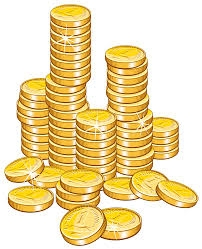Did you find an error or inaccuracy? Feel free to write us. Thank you!Tips to related online calculators
Need help to calculate sum, simplify or multiply fractions? Try our fraction calculator.
Do you have a linear equation or system of equations and looking for its solution? Or do you have a quadratic equation?

## Related math problems and questions:

• Soccer balls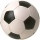Pupils in one class want to buy two soccer balls together. If each of them brings 12.50 euros, they will miss 100 euros; if each brings 16 euros, they will remain 12 euros. How many students are in the class?
• Summerjob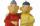Albert, Beny, Cyril and Dan worked on summerjob. From the total revenue Albert received 2/5, Beny 1/6 , 3/10 Cyril and Dan the rest which is 324 euros. How many euros earned every one of them?
• Mr. Brown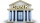Mr. Brown invested 15,000 euros in the bank for an annual term deposit. After the first year, he gained 600 euros. What was the annual interest rate of his deposit?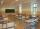The Slovakia circulates a lot of myths, particularly ideas about what must be free. For example - education should be free - children of poor parents should know at least read and write. Calculate how much a student would have to pay per hour of teaching,
• Dog price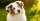Tereza agreed to get a dog and 6000 crowns a year for help in a dachshund breeding station. After eight months, she had to finish work and got a dog and 2000 crowns. What price does a dog have?
• Savings 2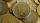Jozef and Michael saved 46 euros together. Michael saved 22 euros more than Jozef. How much did save each of them?
• Salary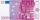Lawyer got to pay 840 Euros in banknotes of 20 and 50 Eur. Total got 18 banknotes. How many was which?
• Class 9.CProfessor collects money in the 9. C for a school trip. 2/3 of the collected amount was from girls and 1/4 from boys. The rest of 410 CZK went from the class fund. How much will the school trip cost in total?
• Columns of two and threeWhen students in one class stand in columns of two, there is none left. When he stands in columns of three, there is one student left. There are 5 more double columns than three columns. How many students are in the class?
• A loanA loan in the amount of \$944 is charged simple interest at an annual rate of 8.1%. How much money is owed 14 months after the loan was made?
• Three friends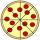Divide 570 euros to three friends so that first will get 50 euros less than the second and third twice more than the first. How many euros will get everyone?
• In the classroomThere are 30 boys and a few girls in the class. In the six months, 28 boys and all girls benefited, which was 95% of all pupils. How many pupils are there in the classroom?
• Four friendsFour friends shared the money. Vasek got 1/4 of the total amount. Tonda received 1/3 of the rest of the money, Joe got a half from the second residue and Jirka left 80. How much money get together?
• DepositOh I total of \$15,000 deposited into simple interest accounts the annual simple interest rate on one account at 6% the annual simple interest rate on the second account at 7% how much should be invested in each account so that the total interest earned is
• Red diplomasThe numbers of students with honors in 2013 and 2014 are in ratio 40:49. How big is the year-on-year percentage increase?
• SavingsThe depositor regularly wants to invest the same amount of money in the financial institution at the beginning of the year and wants to save 10,000 euros at the end of the tenth year. What amount should he deposit if the annual interest rate for the annua
• Money duoJulius and Mark have together 45 euros. Mark has 50% more money than Julius. Determine the amount of money that have Mark and Julius.